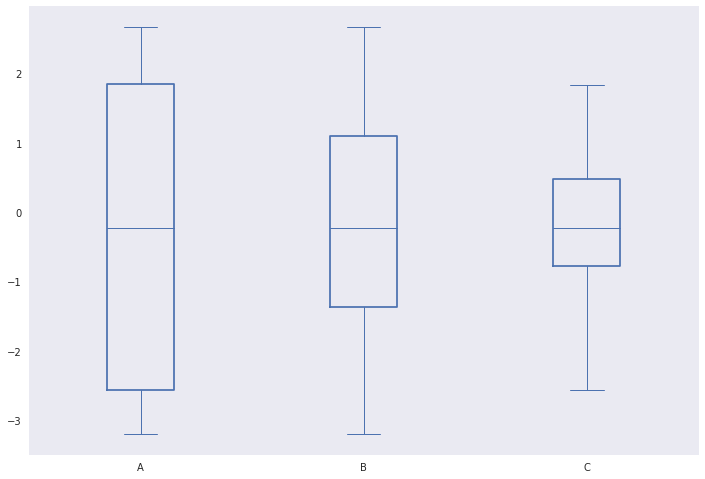# Matplotlib boxplots with custom percentiles

16 Jun 2019

This post was inspired by a question I answered on stack overflow. In the question a user asked if it was possible to make a boxplot with box boundaries at arbitrary percentiles, using matplotlib. Of course, with matplotlib anything is possible and so I set to work…

## Convention

With box and whisker plots it is convention to plot the 25th and 75th percentiles of the data. Thus, one should be aware that departing from this convention comes at a risk of misleading readers. You should also carefully consider what altering the box percentiles means to outlier classification and the whiskers of the boxplot.

## Quick solution

A quick fix (ignoring any implications for whisker locations) is to compute the boxplot statistics we desire, alter the locations of q1 and q3, and then construct the plot with ax.bxp:

import matplotlib.cbook as cbook
import matplotlib.pyplot as plt
import numpy as np

# Generate some random data to visualise
np.random.seed(2019)
data = np.random.normal(size=100)

stats = {}
# Compute the boxplot stats (as in the default matplotlib implementation)
stats['A'] = cbook.boxplot_stats(data, labels='A')
stats['B'] = cbook.boxplot_stats(data, labels='B')
stats['C'] = cbook.boxplot_stats(data, labels='C')

# For box A compute the 1st and 99th percentiles
stats['A']['q1'], stats['A']['q3'] = np.percentile(data, [1, 99])
# For box B compute the 10th and 90th percentiles
stats['B']['q1'], stats['B']['q3'] = np.percentile(data, [10, 90])
# For box C compute the 25th and 75th percentiles (matplotlib default)
stats['C']['q1'], stats['C']['q3'] = np.percentile(data, [25, 75])

fig, ax = plt.subplots(1, 1)
# Plot boxplots from our computed statistics
bp = ax.bxp([stats['A'], stats['B'], stats['C']], positions=range(3))

# Colour the lines in the boxplot blue
for element in bp.keys():
plt.setp(bp[element], color='C0')


However, viewing the plot produced we see that altering q1 and q3 whilst leaving the whiskers unchanged may not be a sensible idea. You could counter this by recomputing eg. stats['A']['iqr'] and the whisker locations stats['A']['whishi'] and stats['A']['whislo'].## A more complete solution

Looking through matplotlib’s source code we find that matplotlib uses matplotlib.cbook.boxplot_stats to compute the statistics used in the boxplot.

Within boxplot_stats we find the code q1, med, q3 = np.percentile(x, [25, 50, 75]). This is the line we can alter to change the plotted percentiles.

So a potential solution would be to make a copy of matplotlib.cbook.boxplot_stats and alter it as we desire. Here I call the function my_boxplot_stats and add an argument percents to make it easy to alter the locations of q1 and q3.

import itertools
from matplotlib.cbook import _reshape_2D
import matplotlib.pyplot as plt
import numpy as np

# Function adapted from matplotlib.cbook
def my_boxplot_stats(X, whis=1.5, bootstrap=None, labels=None,
autorange=False, percents=[25, 75]):

def _bootstrap_median(data, N=5000):
# determine 95% confidence intervals of the median
M = len(data)
percentiles = [2.5, 97.5]

bs_index = np.random.randint(M, size=(N, M))
bsData = data[bs_index]
estimate = np.median(bsData, axis=1, overwrite_input=True)

CI = np.percentile(estimate, percentiles)
return CI

def _compute_conf_interval(data, med, iqr, bootstrap):
if bootstrap is not None:
# Do a bootstrap estimate of notch locations.
# get conf. intervals around median
CI = _bootstrap_median(data, N=bootstrap)
notch_min = CI
notch_max = CI
else:

N = len(data)
notch_min = med - 1.57 * iqr / np.sqrt(N)
notch_max = med + 1.57 * iqr / np.sqrt(N)

return notch_min, notch_max

# output is a list of dicts
bxpstats = []

# convert X to a list of lists
X = _reshape_2D(X, "X")

ncols = len(X)
if labels is None:
labels = itertools.repeat(None)
elif len(labels) != ncols:
raise ValueError("Dimensions of labels and X must be compatible")

input_whis = whis
for ii, (x, label) in enumerate(zip(X, labels)):

# empty dict
stats = {}
if label is not None:
stats['label'] = label

# restore whis to the input values in case it got changed in the loop
whis = input_whis

# note tricksyness, append up here and then mutate below
bxpstats.append(stats)

# if empty, bail
if len(x) == 0:
stats['fliers'] = np.array([])
stats['mean'] = np.nan
stats['med'] = np.nan
stats['q1'] = np.nan
stats['q3'] = np.nan
stats['cilo'] = np.nan
stats['cihi'] = np.nan
stats['whislo'] = np.nan
stats['whishi'] = np.nan
stats['med'] = np.nan
continue

# up-convert to an array, just to be safe
x = np.asarray(x)

# arithmetic mean
stats['mean'] = np.mean(x)

# median
med = np.percentile(x, 50)
## Altered line
q1, q3 = np.percentile(x, (percents, percents))

# interquartile range
stats['iqr'] = q3 - q1
if stats['iqr'] == 0 and autorange:
whis = 'range'

# conf. interval around median
stats['cilo'], stats['cihi'] = _compute_conf_interval(
x, med, stats['iqr'], bootstrap
)

# lowest/highest non-outliers
if np.isscalar(whis):
if np.isreal(whis):
loval = q1 - whis * stats['iqr']
hival = q3 + whis * stats['iqr']
elif whis in ['range', 'limit', 'limits', 'min/max']:
loval = np.min(x)
hival = np.max(x)
else:
raise ValueError('whis must be a float, valid string, or list '
'of percentiles')
else:
loval = np.percentile(x, whis)
hival = np.percentile(x, whis)

# get high extreme
wiskhi = np.compress(x <= hival, x)
if len(wiskhi) == 0 or np.max(wiskhi) < q3:
stats['whishi'] = q3
else:
stats['whishi'] = np.max(wiskhi)

# get low extreme
wisklo = np.compress(x >= loval, x)
if len(wisklo) == 0 or np.min(wisklo) > q1:
stats['whislo'] = q1
else:
stats['whislo'] = np.min(wisklo)

# compute a single array of outliers
stats['fliers'] = np.hstack([
np.compress(x < stats['whislo'], x),
np.compress(x > stats['whishi'], x)
])

# add in the remaining stats
stats['q1'], stats['med'], stats['q3'] = q1, med, q3

return bxpstats


With this in place we can compute our statistics and then plot with plt.bxp.

# Generate some random data to visualise
np.random.seed(2019)
data = np.random.normal(size=100)

stats = {}

# Compute the boxplot stats with our desired percentiles
stats['A'] = my_boxplot_stats(data, labels='A', percents=[1, 99])
stats['B'] = my_boxplot_stats(data, labels='B', percents=[10, 90])
stats['C'] = my_boxplot_stats(data, labels='C', percents=[25, 75])

fig, ax = plt.subplots(1, 1)
# Plot boxplots from our computed statistics
bp = ax.bxp([stats['A'], stats['B'], stats['C']], positions=range(3))

# Colour the lines in the boxplot blue
for element in bp.keys():
plt.setp(bp[element], color='C0')


See that with this solution the whiskers are adjusted in our function based on our selected percentiles: